How to Learn in 24 Hours?The Rapid Learning Movie

 Need Help? M-F: 9am-5pm(PST): Toll-Free: (877) RAPID-10 US Direct: (714) 692-2900 Int'l: 001-714-692-2900 24/7 Online Technical Support: The Rapid Support Center Secure Online Order:Got Questions? Frequently Asked Questions
 Need Proof? Testimonials by Our Users
 Trustlink is a Better Business Bureau Program. Rapid Learning Center is a fivr-star business. External TrustLink Reviews

 Rapid Learning Courses: MCAT in 24 Hours (2015-16) USMLE in 24 Hours (Boards) Chemistry in 24 Hours Biology in 24 Hours Physics in 24 Hours Mathematics in 24 Hours Psychology in 24 Hours SAT in 24 Hours ACT in 24 Hours AP in 24 Hours CLEP in 24 Hours DAT in 24 Hours (Dental) OAT in 24 Hours (Optometry) PCAT in 24 Hours (Pharmacy) Nursing Entrance Exams Certification in 24 Hours eBook - Survival Kits Audiobooks (MP3)

 Tell-A-Friend: Have friends taking science and math courses too? Tell them about our rapid learning system.Home »  Physics »  College Physics

Rotational Motion and Equilibrium

Topic Review on "Title":

A unit of rotational displacement; one revolution equals 2 ∏ radians.

Angular Velocity:

• Also called rotational speed, the number of rotations or revolutions per unit of time, often measured in rpm, revolutions per minute.

Table of rotational motion formulas, and the related linear motion formulas:

 Linear motion formula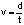Rotational motion formula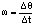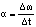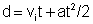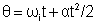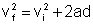Torque:
The rotational quantity that causes rotation; the product of force times lever arm.

Moment of inertia:

• Also called rotational inertia, The rotational equivalent of linear inertia; a measure of the ease of rotating some object.

Newton’s second law for rotational motion:

• Σ Ƭ=I α  The sum of the torques is equal to the rotational inertia times the angular acceleration.

Rotational Equilibrium:

• The situation when the net torque on an object equals zero.

Angular momentum:

• The rotational equivalent of linear momentum that describes the tendency of an object to continue rotating.

Rapid Study Kit for "Title":
 Flash Movie Flash Game Flash Card Core Concept Tutorial Problem Solving Drill Review Cheat Sheet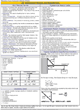"Title" Tutorial Summary : Instead of moving in a straight line, many object rotate.  This rotation can be described with terms and formulas that are analogous to linear motion descriptors. For example, in linear motion a force causes a mass to accelerate.  In rotational motion, a torque causes a mass with some amount of rotational inertia, to exhibit angular acceleration.  The concepts are very similar.  Also, just as an object could be in equilibrium when the forces on it add up to zero, an object can be in rotational equilibrium when the net torque on it is zero.  When studying these new concepts, apply your previous knowledge of linear motion.

 Tutorial Features: Specific Tutorial Features: • Diagrams to illustrate the lever arms, angles, and other quantities encountered in problems. • Animated diagrams to actually show the rotational motion. • Problem-solving techniques are used to work out and illustrate the example problems, step by step. Series Features: • Concept map showing inter-connections of new concepts in this tutorial and those previously introduced. • Definition slides introduce terms as they are needed. • Visual representation of concepts • Animated examples—worked out step by step • A concise summary is given at the conclusion of the tutorial.

 "Title" Topic List: Angular motion compared to linear motion Angular displacement, angle Angular speed Angular acceleration Related formulas Torque Units   Direction Applications Moment of Inertia Description Example Formulas Rotational Equilibrium Angular Momentum Formula Conservation of angular momentum Examples

See all 24 lessons in College Physics, including concept tutorials, problem drills and cheat sheets:
Teach Yourself College Physics Visually in 24 Hours